## How to Delete Numbers after Decimal Point in Excel

This post will guide you how to remove decimals without rounding in Excel. How do I delete numbers after decimal point with a formula in the given cell in Excel.

## Deleting Numbers after Decimal Point

Assuming that you have a list of data in range B1:B5, in which contain decimal values. And you want to remove all decimal without rounding for a given cell in Excel. You can use the TRUNC function to achieve the result. Like this:

`=TRUNC(B1,0)`

Type this formula into a blank cell and press Enter key on your keyboard, and then drag the AutoFill Handle over to other cells to apply this formula.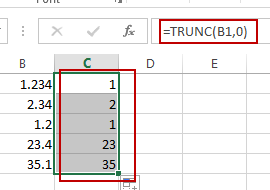You can also use another formula based on the INT function to achieve the same result of deleting numbers after decimal point in Excel. Like this:

`=INT(B1)`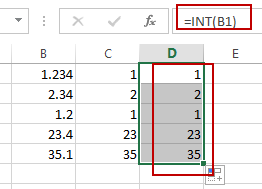## Extract Date from a Date and Time

This post will guide you how to extract date part from a date with time values in Excel. How do I remove time part from a date in Excel. How to get only date part from the date with time values via Format cells feature or Excel function. How to extract date from a date and time with VBA code in Excel.

## Extract date with Format Cells

You can use the Format cells feature to extract date from a date and time value, just do the following steps:

#1 select the cells from which you want to extract date

#2 right click on those cells, select Format Cells… menu from the drop down menu list. And the Format Cells window will appear.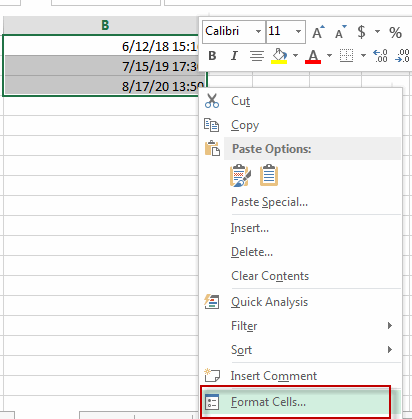#3 switch to Number tab, choose Date category under Category: section. Then select one type of dates such as: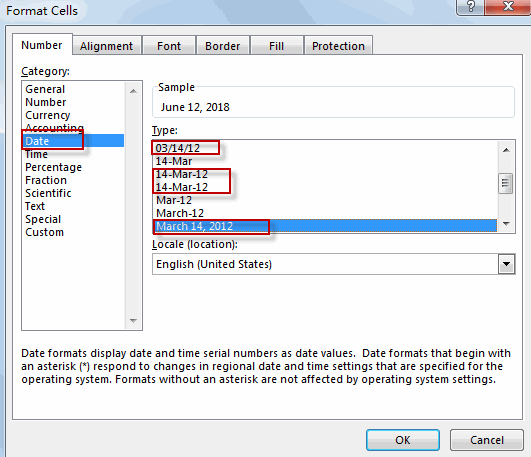#4 click OK button, you will see that the date has been extracted from each date and time value.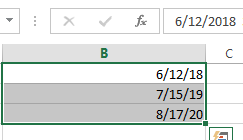## Extract date with Function

The date is represented as serial number and times are fractional values in Excel. So if you want to only display the date part, just using the INT function to remove time portion. Or you can also use the TRUNC function to discard the time portion.

Type the below formula in formula box:

=INT(B1)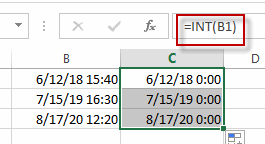Or

=TRUNC(B1)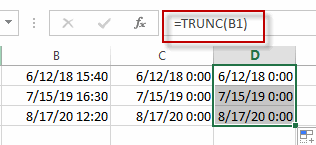Note: you should make sure the date with time values in one cell is a date format.

Extract date with VBA Code

You can also write an Excel VBA Marco to extract date portion from a date with time values in Excel. Just do the following steps:

1# click on “Visual Basic” command under DEVELOPER Tab.2# then the “Visual Basic Editor” window will appear.

3# click “Insert” ->”Module” to create a new module.4# paste the below VBA code into the code window. Then clicking “Save” button.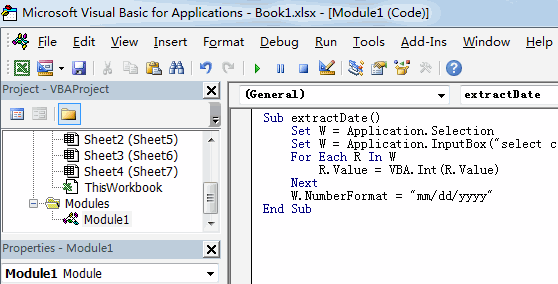```Sub extractDate()
Set W = Application.Selection
Set W = Application.InputBox("select cells of Range:", "extract date from a date and time", W.Address, Type:=8)
For Each R In W
R.Value = VBA.Int(R.Value)
Next
W.NumberFormat = "mm/dd/yyyy"
End Sub```

5# back to the current worksheet, then run the above excel macro. Click Run button.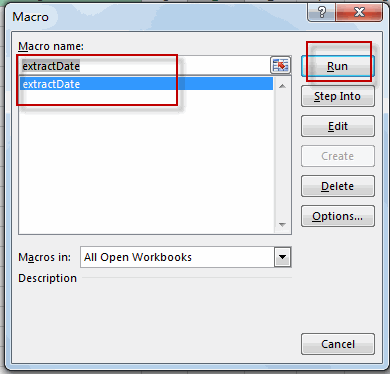6# Select one range from which you want to extract date portion.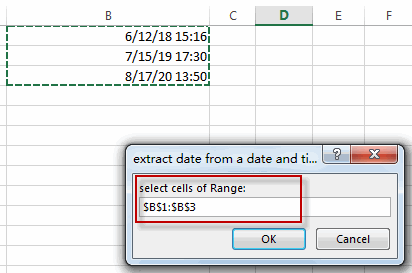#7 Let’s see the result: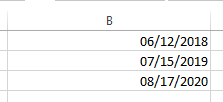### Related Functions

• Excel INT function
The Excel INT function returns the integer portion of a given number. And it will rounds a given number down to the nearest integer.The syntax of the INT function is as below:= INT (number)…
• Excel TRUNC function
The Excel TRUNC function truncates a number to an integer by removing the fractional part of the number.The syntax of the TRUNC function is as below:=TRUNC (number, number_digits)…

## Excel TRUNC Function

This post will guide you how to use Excel TRUNC function with syntax and examples in Microsoft excel.

### Description

The Excel TRUNC function truncates a number to an integer by removing the fractional part of the number. So this function returns a truncated number based on a given number of digits in Excel.

The TRUNC function is a build-in function in Microsoft Excel and it is categorized as a Math and Trigonometry Function.

The TRUNC function is available in Excel 2016, Excel 2013, Excel 2010, Excel 2007, Excel 2003, Excel XP, Excel 2000, Excel 2011 for Mac.

### Syntax

The syntax of the TRUNC function is as below:

`=TRUNC (number, number_digits)`

Where the TRUNC function arguments are:

• number -This is a required argument. The number that you want to truncate.
• number_digits – This is an optional argument. It will specify the number of decimal places to truncate the given number to. If this argument is omitted, and the TRUNC function will set number_digits argument as zero value by default.

Note:

• If the number_digits argument is a positive value, the function will truncate the number of digits to the right of the decimal point.
• If the number_digits argument is equal to 0, then the function will truncate the number to the nearest integer.
• If the number_digits argument is a negative value, the function will truncate the number to the left of the decimal point.
• The TRUNC and INT functions are very similar, they both can return the integer part of a given number. The TRUNC function will remove the fractional part of the number. And the INT function will round numbers down to the nearest integer based on the value of the fractional part of the number. With negative number, the TRUNC function and the INT function are different, as the returned result are different.

### Excel TRUNC Function Examples

The below examples will show you how to use Excel TRUNC Function to truncate a given number to a specified number of decimal places in Excel.

1# to truncate a number 4.8 to return the integer part, enter the following formula in Cell B1.

`=TRUNC(4.8)`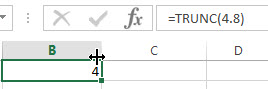2# to truncate a negative number -4.5 to return the integer part, enter the following formula in Cell B2.

`=TRUNC(-4.5)`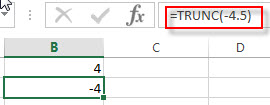3# to truncate a number between 0 and 1 to return the integer part, enter the following formula in Cell B3.

`=TRUNC(0.35)`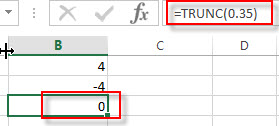### Related Functions

• Excel INT Function
The Excel INT function returns the integer portion of a given number. And it will rounds a given number down to the nearest integer. The syntax of the INT function is as below:= INT (number)…